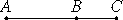# Proposition 24

If an even number is subtracted from an even number, then the remainder is even.

Let the even number BC be subtracted from the even number AB.

I say that the remainder CA is even.VII.Def.6

Since AB is even, therefore it has a half part. For the same reason BC also has a half part, so that the remainder CA also has a half part, and CA is therefore even.

Therefore, if an even number is subtracted from an even number, then the remainder is even.

Q.E.D.

## Guide

This proposition is used in four of the next five propositions.